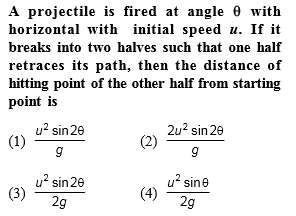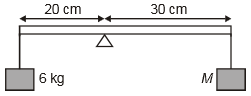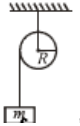A soldier is firing 20 bullets per second from his gun having a muzzle speed of 150 m/s. The mass of each bullet is 50 g. If they strike the wall and rebound with the same speed, then the force on the wall is

1.  75 N

2.  150 N

3.  300 N

4.  600 N

Concept Questions :-

Linear momentum
High Yielding Test Series + Question Bank - NEET 2020

Difficulty Level:The projectile breaks into fragments at the highest point of motion

Concept Questions :-

Torque
High Yielding Test Series + Question Bank - NEET 2020

Difficulty Level:

The value of M, as shown, for which the rod will be in equilibrium is (rod is massless)1.  1 kg

2.  2 kg

3.  4 kg

4.  6 kg

Concept Questions :-

Torque
High Yielding Test Series + Question Bank - NEET 2020

Difficulty Level:

The minimum coefficient of friction for a solid sphere to roll without slipping on an inclined plane of inclination 45$°$ is

1.  2/7

2.  1/3

3.  1/2

4.  2/5

Concept Questions :-

Rolling motion
High Yielding Test Series + Question Bank - NEET 2020

Difficulty Level:

A wheel is rolling along a horizontal ground with a speed o 10 m/s. The magnitude of the velocity of the points at the extremities of the horizontal diameter of the wheel is equal to

(1)

(2) 10 m/s

(3)

(4)

Concept Questions :-

Rolling motion
High Yielding Test Series + Question Bank - NEET 2020

Difficulty Level:

A projectile of mass 50 kg is shot vertically upwards with an initial velocity of 100ms-1. After 5 seconds it explodes into two fragments, one of which having mass 20 kg, travels vertically up with a velocity of 150 ms-1. The velocity of the other fragment at that instant is -          [Take g= 9.8 m/${s}^{2}$]

1.  100 ms-1

2.  150 ms-1

3.  -150 ms-1

4.  -15 ms-1

Concept Questions :-

Linear momentum
High Yielding Test Series + Question Bank - NEET 2020

Difficulty Level:

A sphere of mass m moving with constant velocity hits another sphere of the same mass at rest. If e is the coefficient of restitution. The ratio of their velocities after the collision is

1.  1 + e

2.

3.

4.

Concept Questions :-

Linear momentum
High Yielding Test Series + Question Bank - NEET 2020

Difficulty Level:

Concept Questions :-

Angular momentum
High Yielding Test Series + Question Bank - NEET 2020

Difficulty Level:

A mass M is moving with constant velocity parallel to the X-axis. Its angular momentum w.r.t. origin is:

1.  Zero

2.  Remains constant

3.  goes on increasing

4.  goes on decreasing

Concept Questions :-

Angular momentum
High Yielding Test Series + Question Bank - NEET 2020

Difficulty Level:

A pulley of mass m & radius R is free to rotate about its axis which is horizontal. A string is wound around it and mass m is attached to its free end. When mass m falls through a distance h, its speed at that instant is:1.  proportional to R

2.  proportional to 1/R

3.  proportional to 1/R2

4.  independent of R

Concept Questions :-

Torque